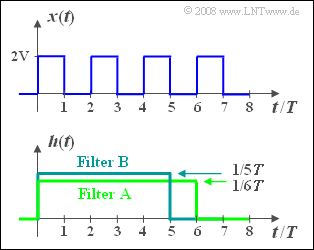# Exercise 1.4Z: Everything RectangularPeriodic rectangular signal and
filter with rectangular impulse response

We consider the periodic rectangular signal  $x(t)$ , whose periodic duration is  $T_0 = 2T$ , according to the sketch above.

• This signal has spectral components at the fundamental frequency  $f_0 = 1/T_0 = 1/(2T)$  and at all odd multiples thereof, that is, at  $3f_0$,  $5f_0,$  and so on. In addition, there is a direct component.
• For this purpose, we consider two filters  $\rm A$  and  $\rm B$  each with rectangular impulse response  $h_{\rm A}(t)$  with duration  $6T$  and  $h_{\rm B}(t)$  with duration  $5T$, respectively.
• The heights of the two impulse responses are such that the areas of the rectangles each add up to  $1$ .

### Questions

1

Compute the output signal  $y_{\rm A}(t)$  of the filter  $\rm A$, in particular the values at  $t = 0$  and  $t = T$.

 $y_{\rm A}(t = 0) \ =\$  $\rm V$ $y_{\rm A}(t = T) \ =\$  $\rm V$

2

Give the absolute value function  $|H_{\rm A}(f)|$ .   What value is obtained at frequency  $f = f_0$?
Interpret the result of the subtask  (1).

 $|H_{\rm A}(f = f_0)| \ =\$

3

Compute the output signal  $y_{\rm B}(t)$  of the filter  $\rm B$, in particular the values at  $t = 0$  and  $t = T$.

 $y_{\rm B}(t = 0) \ =\$  $\rm V$ $y_{\rm B}(t = T) \ =\$  $\rm V$

4

What is the absolute value function  $|H_{\rm B}(f)|$, especially at frequencies  $f = f_0$  and  $f = 3 · f_0$?
Use this to interpret the result of the subtask  (3).

 $|H_{\rm B}(f = f_0)| \ =\$ $|H_{\rm B}(f = 3f_0)| \ =\$

### Solution

#### Solution

(1)  The output signal is the result of the convolution operation between  $x(t)$  and  $h_{\rm A}(t)$:

$$y_{\rm A}(t) = x (t) * h_{\rm A} (t) = \int_{ - \infty }^{ + \infty } {x ( \tau )} \cdot h_{\rm A} ( {t - \tau } ) \hspace{0.1cm}{\rm d}\tau.$$
• Because of the rectangular function and the duration  $6T$  this can also be written as follows:
$$y_{\rm A}(t) = \frac{1}{6T}\cdot \int_{t-6T}^{t}x(\tau)\hspace{0.15cm} {\rm d}\tau.$$
• It can be seen that this equation gives the same result  $y_{\rm A}(t) \rm \underline{\: = 1V}$  for all  $t$ .

(2)  The magnitude of the frequency response is  $|H_{\rm A}(f)| = |{\rm si}(\pi \cdot f \cdot 6T)|.$   This has zeros at an interval of  $1/(6T)$ .

• So, there are also zeros at  $f_0$,  $3f_0$,  $5f_0$  etc., respectively.
• In particular,  $|H_{\rm A}(f = f_0)| \underline{\: = 0}$ holds, too.
• From the spectrum  $X(f)$  only the direct component  $1 \hspace{0.05cm} \rm V$  remains unchanged.
• In contrast to this, all other spectral lines in  $Y_{\rm A}(f)$  are no longer included.

(3)  Analogous to the subtask  (1)  for the output signal the following can be recorded:

$$y_{\rm B}(t) = \frac{1}{5T}\cdot \int_{t-5T}^{t}x(\tau)\hspace{0.15cm} {\rm d}\tau.$$
• This results in a triangular shape fluctuating around the mean  $1 \ \rm V$    ⇒   see bottom graph.
• Since two rectangles and three gaps each are covered by the integration interval, the following holds for  $t = 0, t = 2T,$  etc.:
$$y_{\rm B}(t) = \frac{2\,{\rm V} \cdot 2T }{5T} \hspace{0.15cm}\underline{= 0.8\,{\rm V} =y_{\rm B}(t=0) }.$$
• For  $t = T,\ 3T, \ 5T,$  etc., there are three rectangles and two gaps each to be considered: One obtains:
$$y_{\rm B}(t) \underline{\: = 1.2 \: {\rm V}=y_{\rm B}(t=T)}.$$

(4)  The magnitude function is generally or at frequencies  $f = f_0 = 1/(2T)$  and  $f = 3f_0$:

\begin{align*} |H_{\rm B}(f)| & = |{\rm si}(\pi \cdot f \cdot 5T)|, \\ |H_{\rm B}(f = f_0)| & = |{\rm si}(\pi \frac{5T}{2T})| = |{\rm si}(2.5\pi )| = \frac{1}{2.5 \pi} \hspace{0.15cm}\underline{= 0.127}, \\ |H_{\rm B}(f = 3f_0)| & = |{\rm si}(7.5\pi )| = \frac{1}{7.5 \pi} \hspace{0.15cm}\underline{=0.042}.\end{align*}

Interpretation:

• The spectral components of the rectangular signal at  $f_0, 3f_0,$  etc., although now no longer suppressed, are increasingly attenuated as the frequency increases, in such a way that the rectangular curve is converted into a periodic triangular signal.   The direct component  $(1 \hspace{0.05cm} \rm V)$  remains unchanged here, too.
• Thus, both filters provide the average value of the input signal.   For the signal  $x(t)$  at hand the filter  $\rm A$  is more suitable than the filter  $\rm B$ for the determination of the mean value, because for the former the length of the impulse response is a multiple of the period  $T_0 = 2T$ .
• If this condition – as with the filter  $\rm B$ – is not fulfilled, an error signal (triangular in this example) is still superimposed on the mean value.